# Equivalent Fractions

##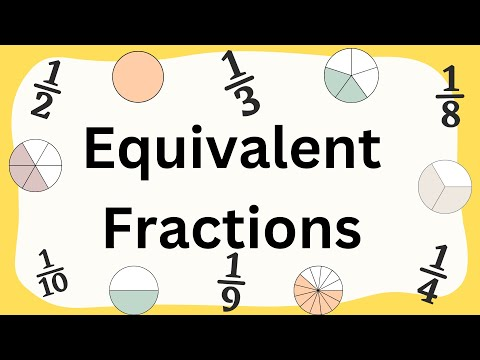By MooMoo Math and Science

defines equivalent fractions# Equivalent Fractions (3.NF.3B)

##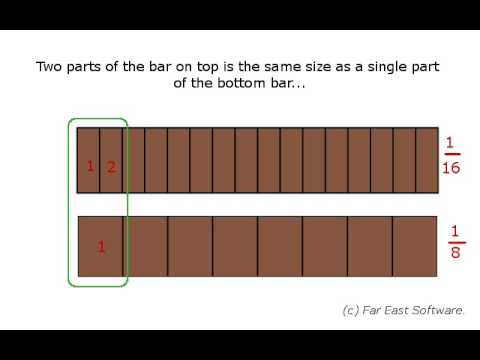By Far East Software

Recognize and generate simple equivalent fractions, e.g., 1/2 = 2/4, 4/6 = 2/3. Explain why the fractions are equivalent, e.g., by using a visual fraction model.# Equivalent Fractions on a Number Line

##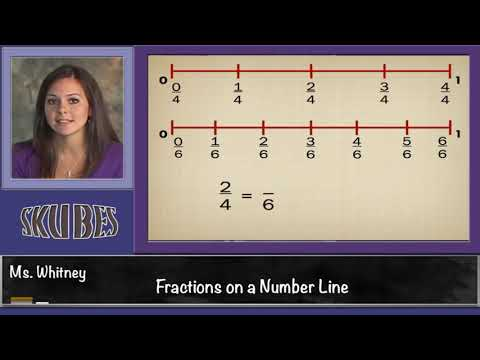By Skubes ed

Explain equivalence of fractions in special cases, and compare fractions by reasoning about their size.# Finding equivalent fractions with visual fraction models

##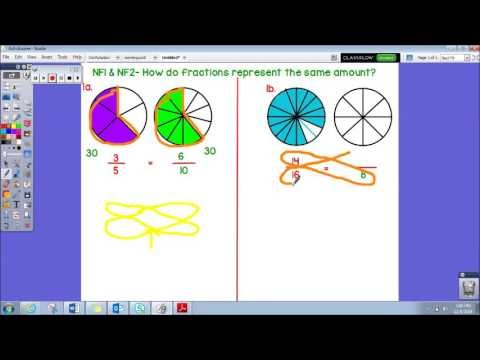By Amy Long

This video explains how to find an equivalent fraction using visual fraction models and also using cross multiplication.# Finding Equivalent Fractions using a Bar Model

##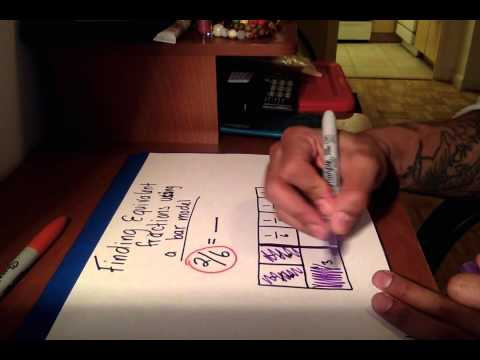By Math with Mr.OK

Explain equivalence of fractions in special cases, and compare fractions by reasoning about their size# Writing Equivalent Fractions with Denominators of 10 and 100

##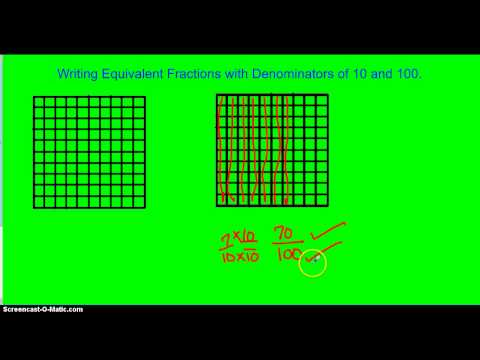By Randykidd

Using Equivalent Fractions to add two fractions with denominators of 10 and 100# Equivalent fractions with visuals

##By Khan Academy

Use same-sized wholes to show equivalent fractions.# [3.NF.3b-1.4] Equivalent Fractions - Common Core Standard

##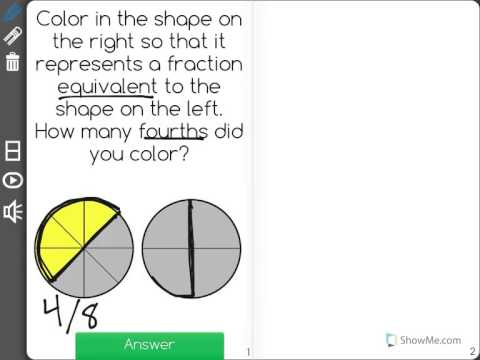By Freckle education

Students must explain why fractions are equivalent using a visual fraction model.# [3.NF.3b-1.0] Equivalent Fractions - Common Core Standard

##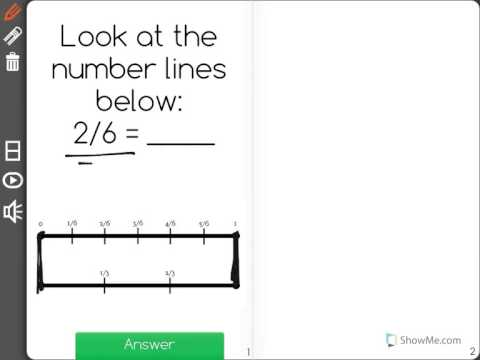By Freckle education

Recognize and generate simple equivalent fractions, e.g., 1/2 = 2/4, 4/6 = 2/3, explaining why the fractions are equivalent.# Visualizing equivalent fractions | Fractions | Pre-Algebra | Khan Academy

##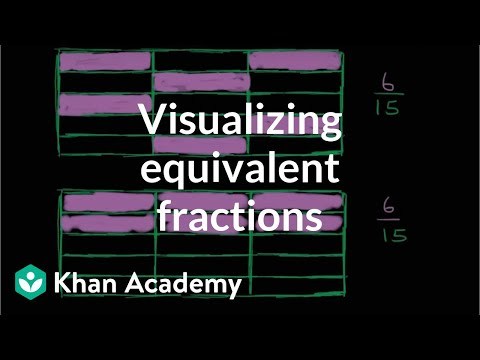By Khan Academy

To understand equivalent fractions it helps to see the fractions using something like a grid with shaded and unshaded sections representing the fraction.# [4.NF.1-1.0] Generate Equivalent Fractions - Common Core Standard

##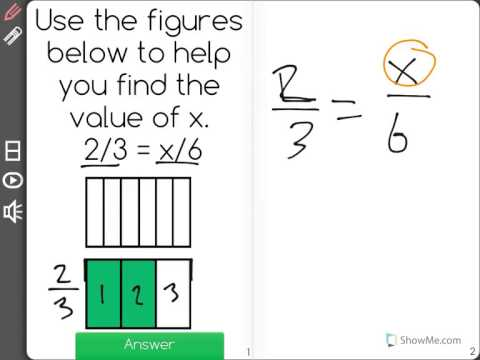By Freckle education

Explain why a fraction a/b is equivalent to a fraction (n x a) / (n x b) by using visual fraction models# Equivalent fractions and different wholes

##By Khan Academy

Learn how two fractions are equivalent only if they refer to the same whole. For example, 1/2 of a small circle is not the same as 1/2 of a big circle.# Equivalent fractions using the number line (3.NF.3A)

##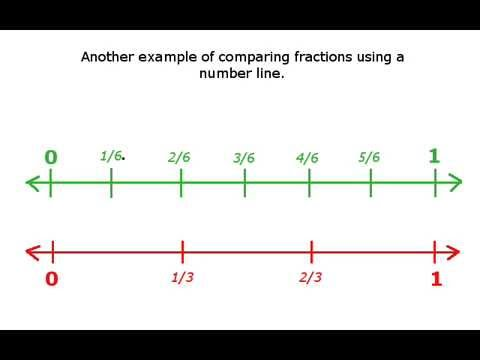By Far East Software

Understand two fractions as equivalent (equal) if they are the same size, or the same point on a number line.# More on equivalent fractions | Fractions | 4th grade | Khan Academy

##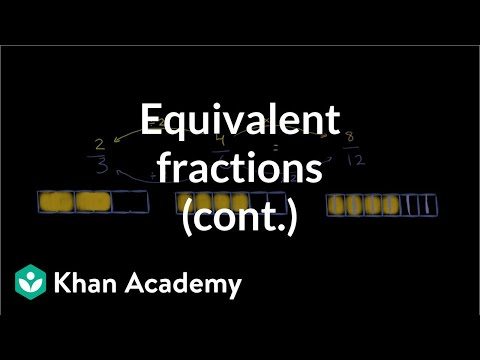By Khan Academy

Important: if you multiply a fraction's numerator and the denominator by the same number, you'll end up with an equivalent fraction. Got it? Maybe? No problem, we'll help!# Equivalent fractions on the number line

##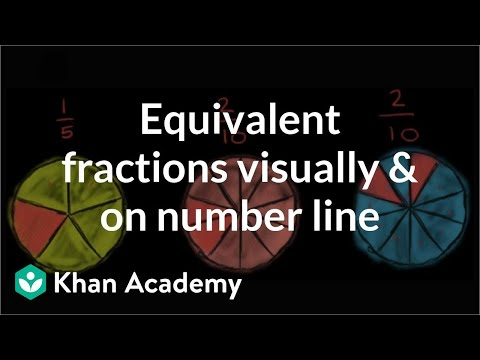By Khan Academy

Use number lines and fraction models to show equivalent fractions.# Equivalent fractions on the number line

##By Khan Academy

Use number lines and fraction models to show equivalent fractions.# Equivalent fractions on the number line

##By Khan Academy

Use number lines and fraction models to show equivalent fractions.# Equivalent fractions on the number line

##By Khan Academy

Create equivalent fractions by dividing a fraction model and number line into smaller parts.# Equivalent fractions on the number line

##By Khan Academy

Use number lines and fraction models to show equivalent fractions.# Equivalent fraction models

##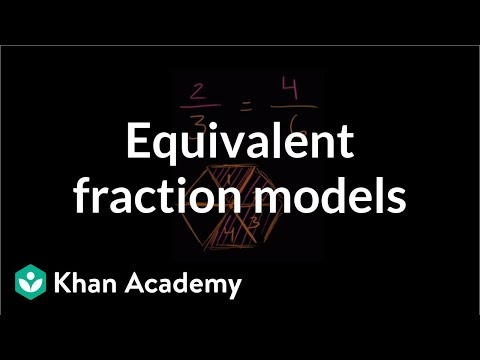By Khan Academy

Use same-sized wholes to show equivalent fractions.